Most Affordable JEE | NEET | 8,9,10 Preparation by Kota's Top IITian Doctor Faculties

# Alternating Current Class 12 Important Questions PhysicsGet important questions of Alternating Current in this question bank for Class 12 Boards exams.View the Physics Question Bank Class 12. These important questions will play significant role in clearing concepts of Physics. This question bank is designed by NCERT keeping in mind and the questions are updated with respect to upcoming Board exams. You will get here all the important questions for class 12 Physics chapter wise CBSE. Learn the concept of alternating current with these important questions of Class 12 point of view. Click Here for Detailed Chapter-wise Notes of PHYSICS for Class 12th, JEE & NEET.

Along with the Claa11 and Class 12 important questions of Alternating Current, notes of other Physics chapters are also available at out website.

Q. At very high frequency of ac a capacitor behaves as a pure conductor. Why ?
Ans. capacitative reactance $X_{C}=1 / \omega C=1 / 2 \pi v C$. At very high frequencies $X_{C}=0$
Q. What are the dimensions of R/L
Ans. $\left[M^{0} L^{0} T^{-1}\right]$
Q. Peak value of emf of an ac source is $E_{0}$. What is its rms value
Ans. rms value of the ac source is $E_{m s}=E_{0} / \sqrt{2}$
Q. In a series LCR circuit, the voltage across an inductor, capacitor and resistor and 20 V, 20 V and 40 V respectively. What is the phase difference between the applied voltage and the current in the circuit.
Ans. Since $V_{L}=V_{C}$ , so circuit is resonant and in resonant condition, RLC series circuit behaves like a resistive circuit. Hence phase difference between voltage and current is $\phi=0^{\circ}$
Q. The number of turns in the secondary coil of a transformer is 10 times that in the primary. What power will be obtained in the secondary if the power applied is 10 W ? Assume 100% efficiency. [NCERT]
Ans. 10 W
Q. (a) Peak voltage of an ac supply is 300 V. What is its rms voltage (b) The rms value of current in an ac circuit is 10 A. What is the peak current [NCERT]
Ans.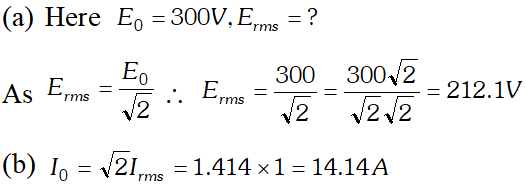Q. A 60 uF capacitor is connected to a $110 \mathrm{V}, 60 \mathrm{Hz}$ ac supply. Determine the rms value of current in $\mathrm{n}$ in the circuit [NCERT]
Ans.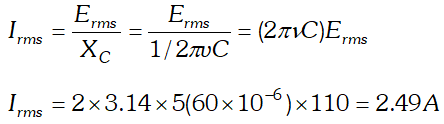Q. Can the voltage drop across the inductor in a series LCR circuit be greater than the applied voltage of the ac source
Ans. Yes, the voltage drops across the inductor or the capacitor in a series circuit can be grater thenthe applied voltage. This is because these voltages are not in phase and they cannot be added like ordinary numbers.
Q. Distinguish between resistance reactance and impedance.
Ans. The resistance is the opposition offered by a resistor R to the flow of ac in a circuit, the reactance is the opposition offered by an inductor L or by a capacitor C or by both while impedance is the opposition offered by L-R, C-R or L-C-R circuits. The resistance is independent of the frequency while the reactance and the impedance do depend on the frequency of ac
Q. Calculate the rms value of the alternating current represented in this figure.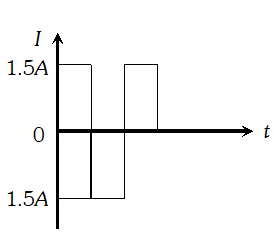Ans. It is clear from the figure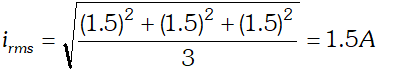Q. An inductor L of reactance XL, is connected in series with a bulb B to an ac source as shown in figure. Briefly explain how does the brightness of the bulb change, when (i) Number of turns of the inductor is reduced and (ii) A capacitor of reactance XC=XL is included in series in the same circuit.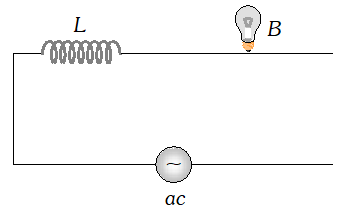Ans. (i) On reducing the number of turns in the inductor, the inductance $L\left(\propto N^{2}\right)$ and hence the inductive reactance $X_{L}(=\omega L)$ decreases. So, the current in the circuit increases and the bulbs glows more brightly. (ii) When $X_{L}=X_{C}$ the impedance of the circuit is minimum (equal to R). Hence the current is maximum and the bulb glows with maximum brightness
Q. A capacitor and a resistor are connected in series with an ac source. If the potential difference across C,R are 120 V, 90 V respectively and if the rms current of the circuit is 3 A, calculate the (i) Impedance, (ii) Power factor of the circuit
Ans.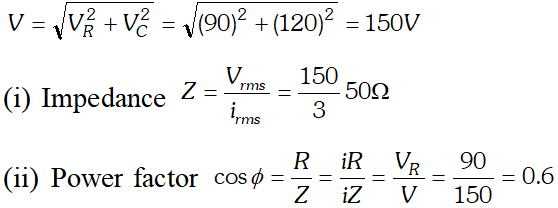Q. A 100 W resistor is connected to a 220 V, 50 Hz ac supply. (a) What is the rms value of current in the circuit? (b) What is the net power onsumed over a full circuit [NCERT]
Ans.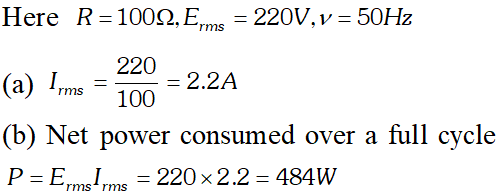Q. A 44 m H inductor is connected to 220 V, 50 Hz ac supply. Determine the rms value of curren in the circuit [NCERT]
Ans.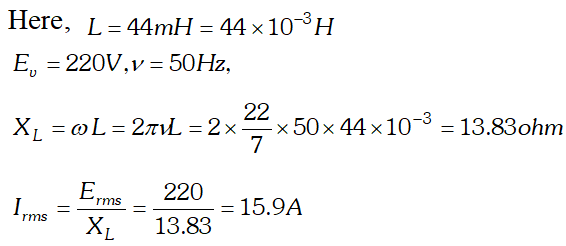Q. Obtain the resonant frequency (wr) of a series LCR circuit with L = 2.0 H, C = 32mF and R = 10 ohm. What is the Q value of this circuit [NCERT]
Ans.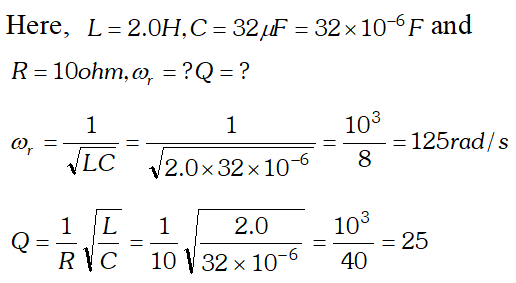Q. A charged 30 $\mu F$capacitor is connected to a 27 mH inductor. What is the angular frequency of free oscillations of the circuit. [NCERT]
Ans.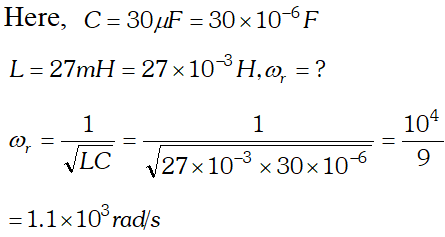Q. Suppose the initial charge on the capacitor in the above question is 6 mC. What is the total energy stored in the capacitor initially ? What is the total energy at later time? [NCERT]
Ans.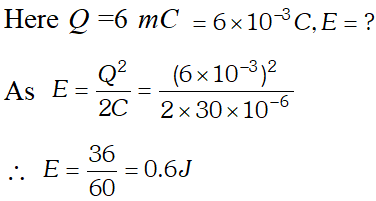At later times, energy is shared between C and L but total energy remains the same, assuming that there is no loss of energy.
Q. A series LCR circuit with $R=20 \Omega, \mathrm{L}=1.5$ Hand $C=35 \mu F$ is connected to a variable frequency 200 V ac supply. When the frequency of the supply equals the natural frequency of the circuit, what is the average power transferred to the circuit in one complete cycle? [NCERT]
Ans. When the frequency of the supply equals the natural frequency of the circuit, resonance occurs.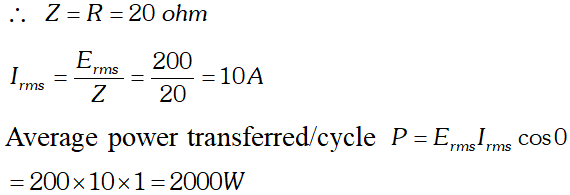Q. An ac source $E=E_{0}$sin $\omega t$ is a applied across an inductor of inductance L. Show mathematically that the current lags the voltage by a phase angle of $\pi / 2$. What is an inductive reactance
Ans. The emf applied across the inductance L is $E=E_{0}$ $\sin \omega t$ If $\frac{d i}{d t}$ rate of change of current through L at any instant, then induced emf in the inductor at the same instant is $e=-L \frac{d i}{d t}$ To maintain the flow of current in this circuit, applied voltage must be equal and opposite to the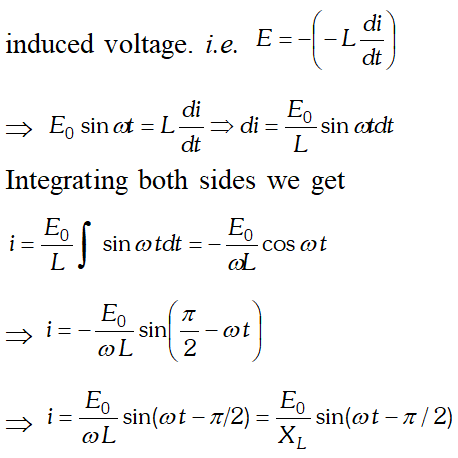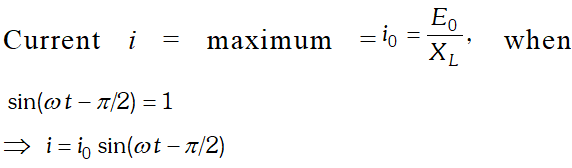Q. Prove that an ideal inductor does not dissipate power in an ac circuit.
Ans. When a source of ac is connected to an inductor L, current in it grows from zero to maximum steady value $I_{0}$ An induced emf developes in the inductor, which opposes the growth of current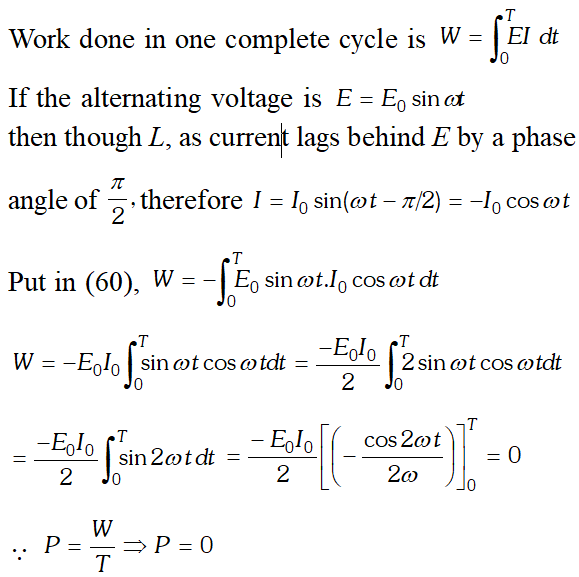Q. Show mathematically that the average power supplied to an ideal capacitor by a source over a complete cycle of ac is zero.
Ans. Suppose an alternating emf $E=E_{0} \sin \omega t$ is applied across an ideal capacitor then current flows through the circuit is $i=i_{0} \sin (\omega t+\pi / 2$ $(\because$In purely capacitive circuits, current leads the voltage by 90°) Hence, work done over a complete cycle of ac is Hence, work done over a complete cycle of ac is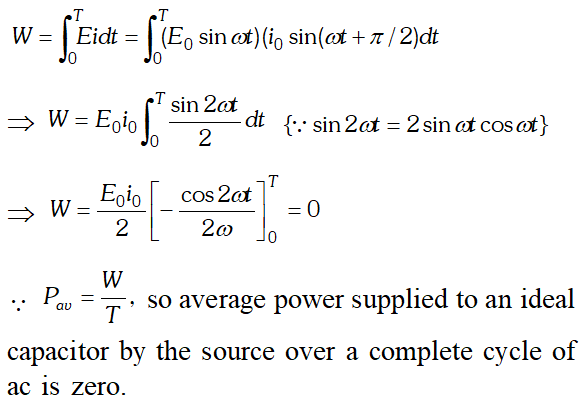Q. Define the term impedance of series LCR circuit. Derive a mathematical expression for it using phasor diagram.
Ans. The opposition offered by series RLC circuit to the flow of current through it is called impedance of the circuit. It is denoted by Z. Suppose a resistance R, inductor L and capacitor of capacitance C are connected in series to an alternating voltage supply $V=V_{0} \sin \omega t$ as shown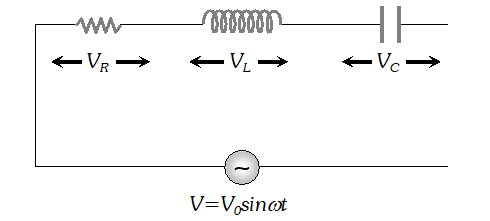The voltage across resistance R is given by $V_{R}=i R$ it is in phase with current and it is denoted by vector $\overrightarrow{\mathrm{OA}}$ (ii) The voltage across inductor L is given by $V_{L}=i X_{L}$ it leads the current by $90^{\circ}$and it is represented by vector $\overrightarrow{O B}$ along Y (iii) The voltage across capacitor C is given by (iii) The voltage across capacitor C is given by it lag behind the current by 90°, and it is represented by along it lag behind the current by $90^{\circ}$, and it is represented by $\overrightarrow{O C}$ along $Y^{\prime}$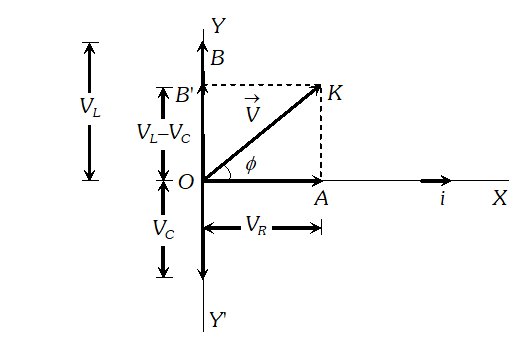As the voltage across $L$ and $C$ have phase difference by $180^{\circ}$ the voltage across $L C .$ Combination is $\left(V_{L}-V_{c}\right),$ assuming that $V_{L}>V_{C}$ It is represented by vector $O B$ in phasor diagram the resultant of $\frac{1}{O A}$ and $\overline{O B}$ is along $\overline{O K}$ hence from phasor diagram it is clear that $|$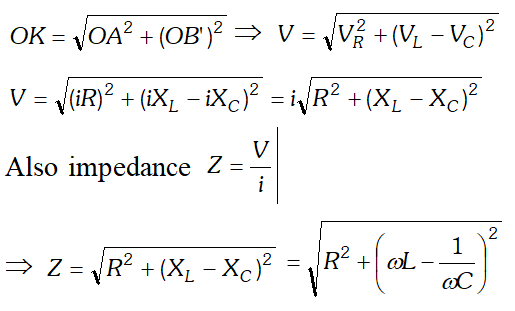Q. What are the causes of loss in transformer ? How they are minimised?
Ans. A transformer have following losses. Copper loss : This loss is due to heating of primary and secondary copper coils of transformer. This loss can be minimised by using thick Cu wires in windings. Iron loss : When a changing magnetic flux is linked with the iron core of the transformer, eddy currents are set up in it. These eddy currents produces heat energy (i.e. loss of energy). The energy loss is minimised by using laminated iron cores. Hysteresis loss : This loss occurs due to the repeated magnetisitaion and demagnetisation of iron core when ac is fed to it. This loss can be minimised by using a core of material like soft iron which has a low hysthersis loss. Magnetic flux leakage : Rate of change of magentic flux linked with the primary coil may not be exactly equal to the rate of change of magnetic flux linked with the secondary coil. It can be minimised by winding the secondary coil inside the primary coil. Vibration of core : A transformer produces humming noise due to magnetisation effect. Some energy is lost in the form of mechanical energy to produce vibration in the core.
Q. A radio can tune over the frequency range of a portion of MW broadcast band (800 kHz to 1200 kHz.) If its LC circuit has an effective inductance of $200 \mu \mathrm{H}$, What must be the range of its variable capacitor [NCERT]
Ans.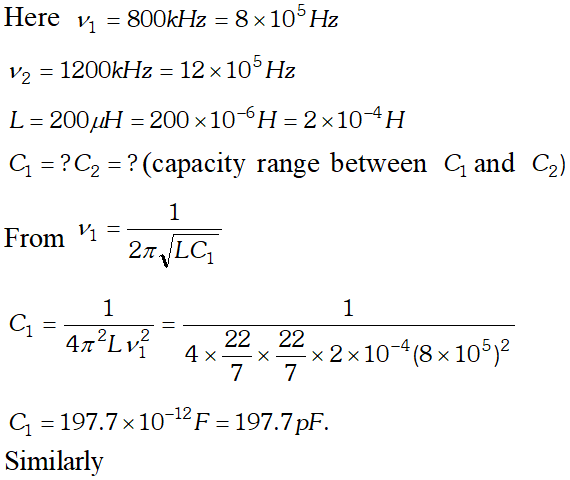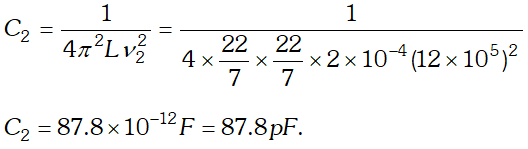Q. Figure given below shows how the reactance of a capacitor varies with frequency. (i) Use the information on graph to calculate the value of capacity of the capacitor (ii) An inductor of inductance L has the same reactance as the capacitor at 100 Hz. Find the value of L. (iii) Using the same axes, draw a graph of reactance against frequency for the inductor given in part (ii). (iv) If this capacitor and inductor were connected in series to a resistor of $10 \Omega$what

would be the impedance of the combination at 300 Hz.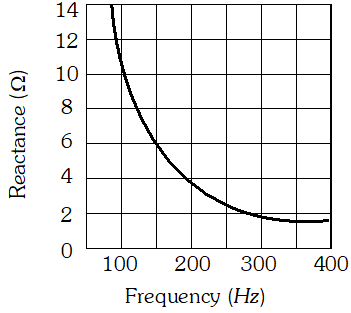Ans.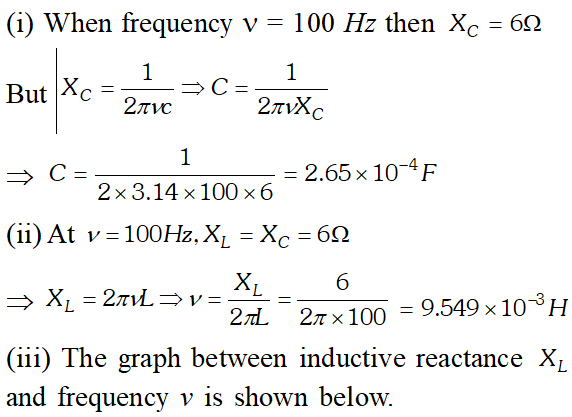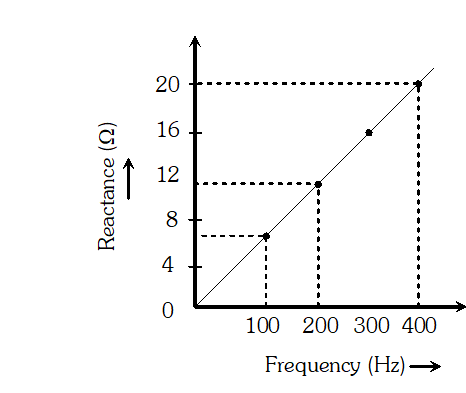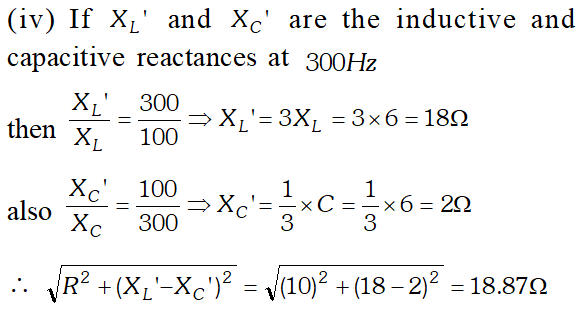Q. The given circuit diagram shows a series LCR circuit connected to a variable frequency 230 V source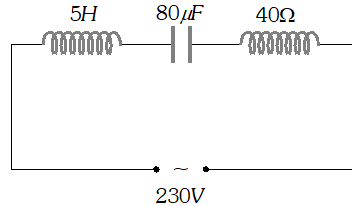(a) Determine the source frequency which drives the circuit in resonance. (b) Obtain the impedance of the circuit and the amplitude of current at the resonating frequency. (c) Determine the rms potential drops across the three elements of the circuit (d) How do you explain the observation that the algebraic sum of the voltages across the three elements obtained in (c) is greater than the supplied voltage
Ans. (i) Source frequency in resonance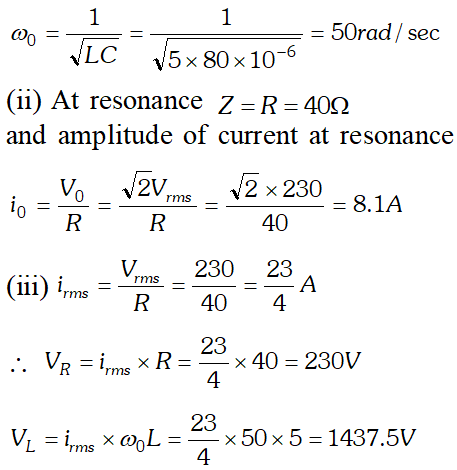In resonance $V_{C}=V_{L}=1437.5 \mathrm{V}$ The algebraic sum of the three voltages is more than the source voltage of 230 V. These voltages are not in the same phase and cannot be added like ordinary numbers. The voltage across L and C are out of phase and get added to zero. So $\left(V_{R}\right)_{m s}=$ applied rms voltage.
Q. The primary coil of an ideal step up transformer has 100 turns and the transformation ratio is also 100. The input voltage the power are 220 V and 1100 W respectively. Calculate. (i) Number of turns in the secondary (ii) the current in the primary (iii) Voltage across the secondary (iv) The current in the secondary (v) power in the secondary
Ans.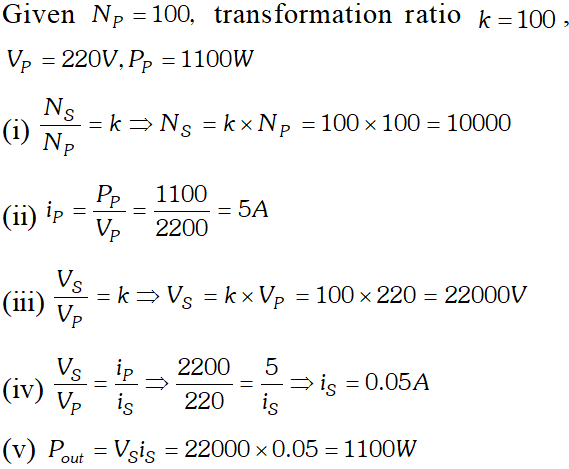Click Here for Detailed Notes of any chapter.

eSaral provides you complete edge to prepare for Board and Competitive Exams like JEE, NEET, BITSAT, etc. We have transformed classroom in such a way that a student can study anytime anywhere. With the help of AI we have made the learning Personalized, adaptive and accessible for each and every one. Visit eSaral Website to download or view free study material for JEE & NEET. Also get to know about the strategies to Crack Exam in limited time period.